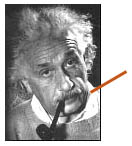The Standard Model as a theory

While the Standard Model provides a very good description of phenomena observed by experiments, it is still an incomplete theory. The problem is that the Standard Model cannot explain why some particles exist as they do. For example, even though physicists knew the masses of all the quarks except for top quark for many years, they were simply unable to accurately predict the top quark's mass without experimental evidence because the Standard Model lacks any explanation for a possible pattern for particle masses.

### Does this mean that the Standard Model is wrong?

No -- but we need to go beyond the Standard Model in the same way that Einstein's Theory of Relativity extended Newton's laws of mechanics. Isaac Newton's laws of mechanics are not wrong, per se, but his theory only works as long as velocity is much smaller than the speed of light. Einstein expanded Newtonian physics with his Theory of Relativity, which allows for the possibility of very high velocities. We will need to extend the Standard Model with something totally new in order to thoroughly explain mass, gravity and other phenomena.I wonder what we'll decide to call the new theory that replaces the Standard Model! The Super-Standard Model? The New Revised Standard Model? The Very Standard Model?